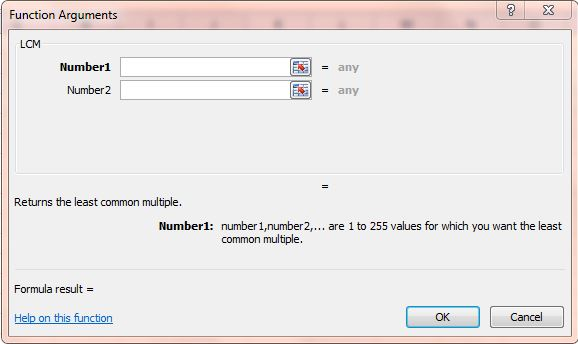# How to use LCM function in Excel spreadsheets [Tip]

LCM (Least Common Multiple) is an Excel function that returns the least common multiple of values. The least common multiple is the lowest integer (a number without fractional component) that is a multiple of all the selected numbers. For example, the lowest common multiple of two and five is 10.

You can add this function to spreadsheets by entering values in the cells or directly into the function’s window. As an example, enter the values 3 and 7 in a couple of alternative cells. Then select a cell to add the LCM function to, and click the fx button. Select LCM to open the function’s window below.Press the button beside the Number1 field to select the function’s values. Instead of selecting one cell value, select both the cells you entered the numbers in for the Number1 field. Then click OK to close the LCM window.The function cell will return a value of 21. Three x seven amounts to 21. Thus, that’s the least common multiple for both the numbers.

You could also enter this function directly into the formulae bar without any spreadsheet data. Select a cell and click the formula bar to input the function. Enter =LCM(3,7) into the formulae bar and press Enter. That will return the least common multiple much the same.

This is a good function for adding fractions with alternative denominators. Check out this video for further details on how to add LCM to Excel spreadsheets.